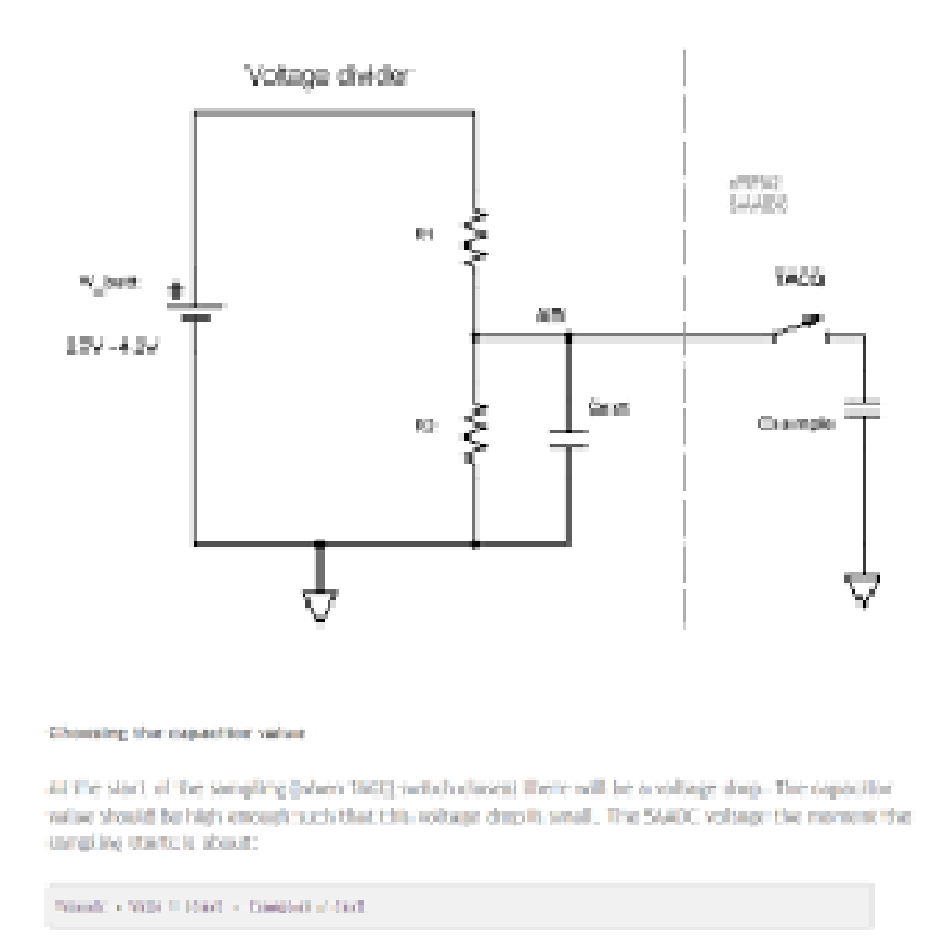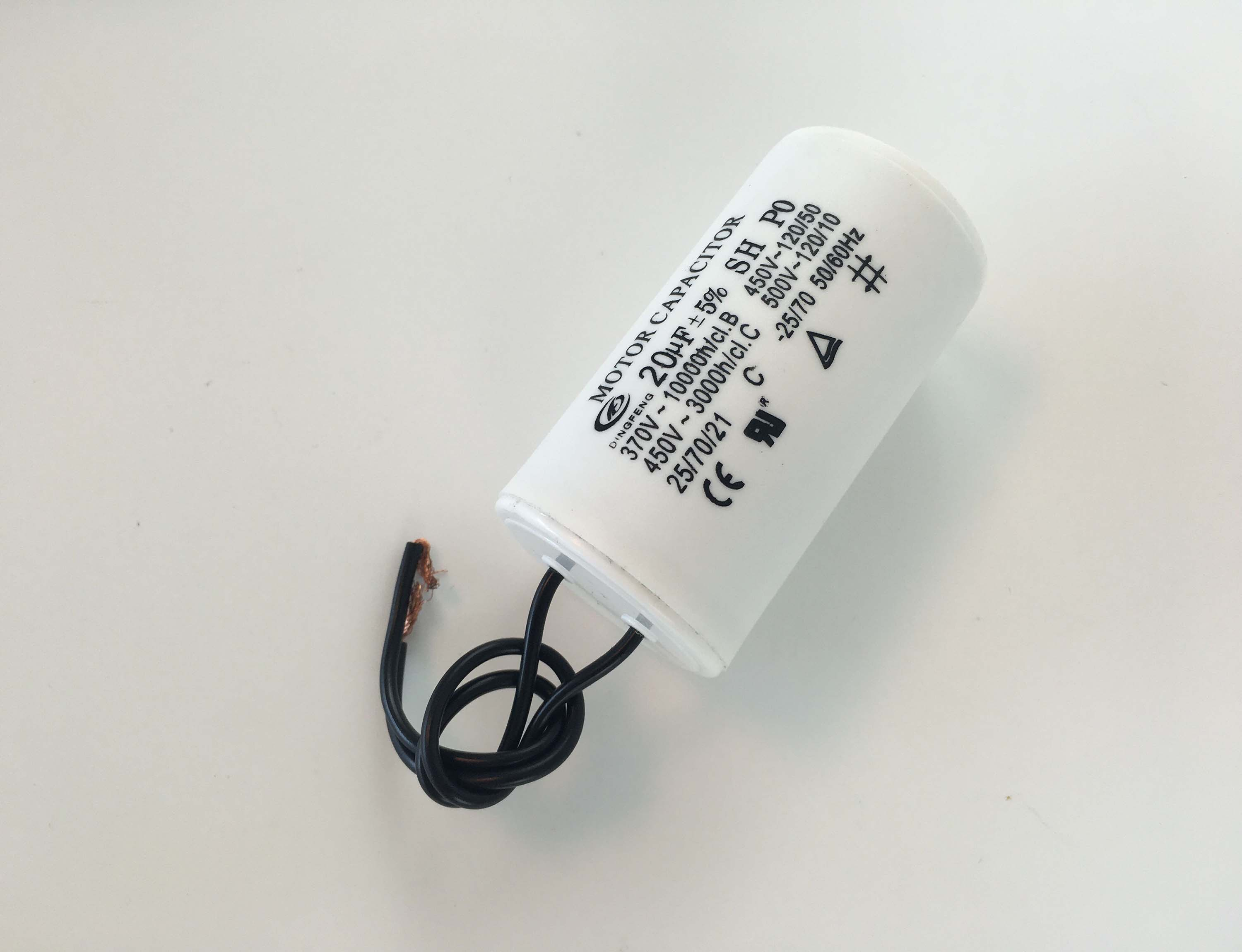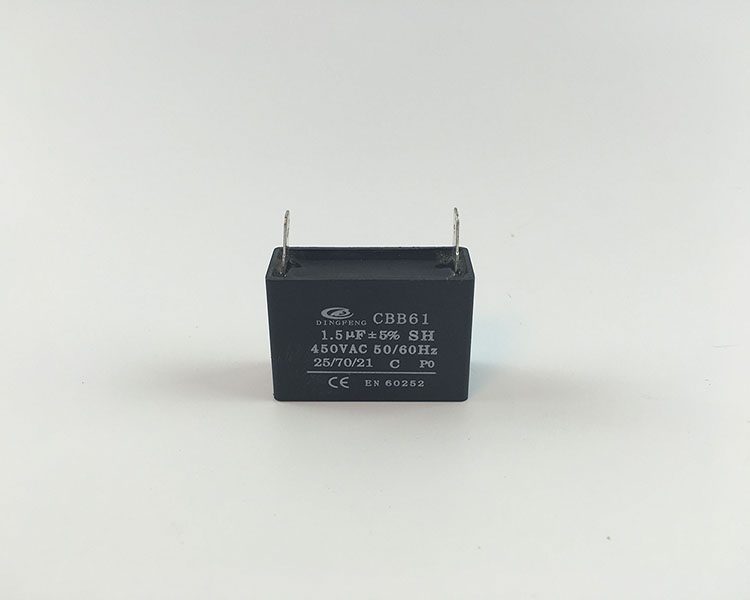Taizhou Dingfeng Electric Appliance Co.,Ltd

## Dingfeng Capacitor---What does the rated voltage of the capacitor mean?

publisher: Yani
Time: 2018-04-09What does the rated voltage of the capacitor mean?

The rated voltage of the capacitor is the maximum voltage that a capacitor can safely access and store.

Remember that capacitors are storage devices. Capacitors store X charge at X voltage; meaning, they hold a certain size charge (1.5µF, 10µF, 50µF, etc.) at a certain voltage (250v,450v,etc.). So when choosing a capacitor you just need to know what size charge you want and at which voltage.

Why do capacitors have different voltage levels? Because you might need different voltages depending on the circuit you're dealing with. Remember, the voltage that a capacitor provides to a circuit is like a battery. The only difference is that the voltage on the capacitor is much faster than the battery, but it is the same concept on how to supply voltage to the circuit.For a circuit, it may require 12 volts, so a capacitor with a rated voltage of 12V or above is required.Take note that a capacitor's voltage rating is not the voltage that the capacitor will charge up to, but only the maximum amount of voltage that a capacitor should be exposed to and can store safely.Keep in mind that a good rule for choosing the voltage ratings for capacitors is not to choose the exact voltage rating that the power supply will supply it. It is normally recommended to give a good amount of room when choosing the voltage rating of a capacitor. You can't really go wrong choosing a higher voltage-rated capacitor than the voltage that the power supply will supply it, but you can definitely go wrong choosing a lower voltage-rated capacitor than the voltage that it will be exposed to. If you charge up a capacitor with a lower voltage rating than the voltage that the power supply will supply it, you risk the chance of the capacitor exploding and becoming defective and unusable. So don't expose a capacitor to a higher voltage than its voltage rating. The voltage rating is the maximum voltage that a capacitor is meant to be exposed to and can store. Some say a good engineering practice is to choose a capacitor that has double the voltage rating than the power supply voltage you will use to charge it. So if a capacitor is going to be exposed to 25 volts, to be on the safe side, it's best to use a 50 volt-rated capacitor.